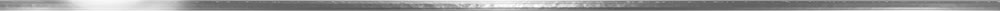# SI RADIATION MEASUREMENT UNITS: CONVERSION FACTORS

A: Since nuclear radiation affects people, we must be able to measure its presence. We also need to relate the amount of radiation received by the body to its physiological effects. Two terms used to relate the amount of radiation received by the body are exposure and dose. When you are exposed to radiation, your body absorbs a dose of radiation.

As in most measurement quantities, certain units are used to properly express the measurement. For radiation measurements they are:

* Roentgen: The roentgen measures the energy produced by gamma radiation in a cubic centimeter of air. It is usually abbreviated with the capital letter "R". A milliroentgen, or "mR", is equal to one one-thousandth of a roentgen. An exposure of 50 roentgens would be written "50 R".

* Rad: Or, Radiation Absorbed Dose recognizes that different materials that receive the same exposure may not absorb the same amount of energy. A rad measures the amount of radiation energy transferred to some mass of material, typically humans. One roentgen of gamma radiation exposure results in about one rad of absorbed dose.

* Rem: Or, Roentgen Equivalent Man is a unit that relates the dose of any radiation to the biological effect of that dose. To relate the absorbed dose of specific types of radiation to their biological effect, a "quality factor" must be multiplied by the dose in rad, which then shows the dose in rems. For gamma rays and beta particles, 1 rad of exposure results in 1 rem of dose.

Other measurement terms: Standard International (SI) units which may be used in place of the rem and the rad are the sievert (Sv) and the gray (Gy). These units are related as follows: 1Sv = 100 rem, 1Gy = 100 rad. Two other terms which refer to the rate of radioactive decay of a radioactive material are curie (Ci) and becquerel (Bq).

Bottom Line: Fortunately, cutting through the above confusion, for purposes of practical radiation protection in humans, most experts agree (including FEMA Emergency Management Institute) that Roentgen, Rad and Rem can all be considered equivalent. The exposure rates you'll usually see will be expressed simply in terms of roentgen (R) or milliroentgen (mR).